The radius of curvature is given by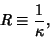(1)

where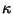is the Curvature. At a given point on a curve,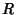is the radius of the Osculating Circle. The symbol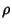is sometimes used instead ofto denote the radius of curvature.

Letandbe given parametrically by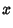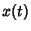(2)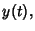(3)

then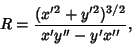(4)

where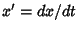and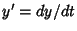. Similarly, if the curve is written in the form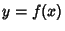, then the radius of curvature is given by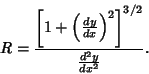(5)